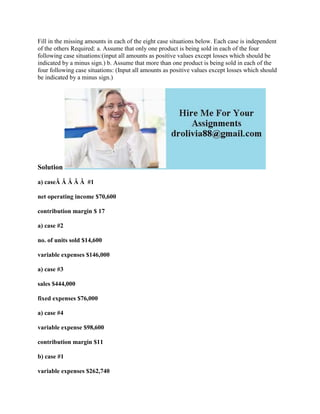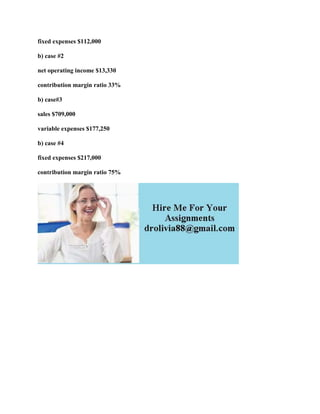Diese Präsentation wurde erfolgreich gemeldet.

# Fill in the missing amounts in each of the eight case situations below.docx

Anzeige
Anzeige
Anzeige
Anzeige
Anzeige
Anzeige
Anzeige
Anzeige
Anzeige
Anzeige
Anzeige×

1 von 2 Anzeige

# Fill in the missing amounts in each of the eight case situations below.docx

Fill in the missing amounts in each of the eight case situations below. Each case is independent of the others Required: a. Assume that only one product is being sold in each of the four following case situations:(input all amounts as positive values except losses which should be indicated by a minus sign.) b. Assume that more than one product is being sold in each of the four following case situations: (Input all amounts as positive values except losses which should be indicated by a minus sign.)
Solution
a) caseÂ Â Â Â Â  #1
net operating income \$70,600
contribution margin \$ 17
a) case #2
no. of units sold \$14,600
variable expenses \$146,000
a) case #3
sales \$444,000
fixed expenses \$76,000
a) case #4
variable expense \$98,600
contribution margin \$11
b) case #1
variable expenses \$262,740
fixed expenses \$112,000
b) case #2
net operating income \$13,330
contribution margin ratio 33%
b) case#3
sales \$709,000
variable expenses \$177,250
b) case #4
fixed expenses \$217,000
contribution margin ratio 75%
.

Fill in the missing amounts in each of the eight case situations below. Each case is independent of the others Required: a. Assume that only one product is being sold in each of the four following case situations:(input all amounts as positive values except losses which should be indicated by a minus sign.) b. Assume that more than one product is being sold in each of the four following case situations: (Input all amounts as positive values except losses which should be indicated by a minus sign.)
Solution
a) caseÂ Â Â Â Â  #1
net operating income \$70,600
contribution margin \$ 17
a) case #2
no. of units sold \$14,600
variable expenses \$146,000
a) case #3
sales \$444,000
fixed expenses \$76,000
a) case #4
variable expense \$98,600
contribution margin \$11
b) case #1
variable expenses \$262,740
fixed expenses \$112,000
b) case #2
net operating income \$13,330
contribution margin ratio 33%
b) case#3
sales \$709,000
variable expenses \$177,250
b) case #4
fixed expenses \$217,000
contribution margin ratio 75%
.

Anzeige
Anzeige

### Fill in the missing amounts in each of the eight case situations below.docx

1. 1. Fill in the missing amounts in each of the eight case situations below. Each case is independent of the others Required: a. Assume that only one product is being sold in each of the four following case situations:(input all amounts as positive values except losses which should be indicated by a minus sign.) b. Assume that more than one product is being sold in each of the four following case situations: (Input all amounts as positive values except losses which should be indicated by a minus sign.) Solution a) caseÂ Â Â Â Â #1 net operating income \$70,600 contribution margin \$ 17 a) case #2 no. of units sold \$14,600 variable expenses \$146,000 a) case #3 sales \$444,000 fixed expenses \$76,000 a) case #4 variable expense \$98,600 contribution margin \$11 b) case #1 variable expenses \$262,740
2. 2. fixed expenses \$112,000 b) case #2 net operating income \$13,330 contribution margin ratio 33% b) case#3 sales \$709,000 variable expenses \$177,250 b) case #4 fixed expenses \$217,000 contribution margin ratio 75%